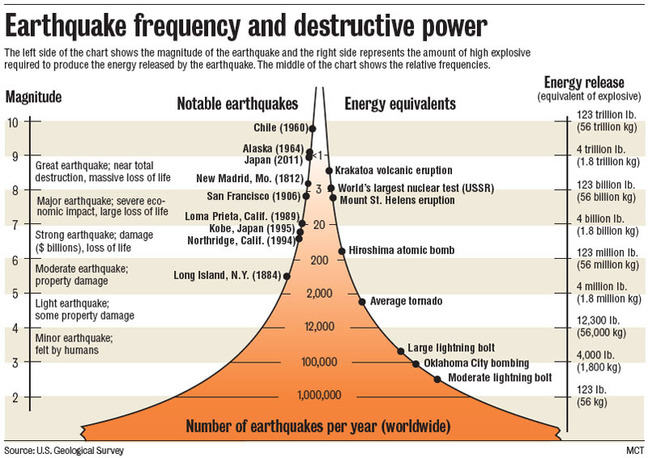2.8

What is a megaquake?

So far we’ve considered earthquakes, but what about the most extreme version, so called megaquakes.

In this course we define extreme events by how frequent they have occurred relative to some timescale (i.e. the age of the Earth).

There is a relationship between the magnitude or size of an earthquake and how frequently it occurs. Earthquakes with the largest magnitudes occur infrequently.

So extreme earthquakes or megaquakes, can be defined as being rare and of the largest magnitudes.

Measuring earthquake magnitude

There are a number of ways to measure the magnitude or size of an earthquake. The most well-known measure is the Richter scale. Scientists now consider this type of scale as outdated.

These days, the USGS and many other agencies report earthquakes using the Moment magnitude scale.

This scale is based on the type and strength of shaking of the earthquake, as measured by seismometers.

Using this measure, we can actually categorise all earthquakes and relate them to how frequently occurs.

This relationship is highlighted in the figure below.Earthquake magnitudes and energy release by Gavin Hayes, USGS, Public Domain.

Defining megaquakes

There is no formal definition of a megaquake. The reason for this is that we are dealing with a continuous scale and not a discrete scale.

This means that any magnitude between 0 and 10 can occur, and that the size of an earthquake does not jump in magnitude from for example 5 to 9.

So choosing a boundary between what is regular and what is extreme, is slightly arbitrary in the case of earthquakes.

But, we would suggest that earthquakes with a magnitude larger than 8 can be considered as megaquakes.

Based on this definition, there is about one megaquake every year.

When and where was the last megaquake to occur?